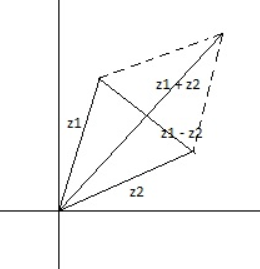QuestionAnswers

# Let z1 and z2 be two complex numbers such that z1 $\ne$ z2 and |z1| = |z2|. If z1​ has positive real part and z2​ has negative imaginary part, then $\dfrac{{{z}_{1}}+{{z}_{2}}}{{{z}_{1}}-{{z}_{2}}}$ may be which of the following:(a) Purely imaginary(b) Real and positive(c) Real and negative(d) None of theseHint: Consider vectors along the position vector of z1​ and z2​ in the Argand Plane and with magnitude equal to |z1| and |z2|. A rhombus will be formed as shown in the figure with z1 + z2 and z1 - z2 in the directions of the diagonals. Use the fact that the diagonals of a rhombus are perpendicular to each other to get z1 + z2 and z1 - z2 will be perpendicular to each other. This means that $\dfrac{{{z}_{1}}+{{z}_{2}}}{{{z}_{1}}-{{z}_{2}}}$ will have an argument of $\pm \dfrac{\pi }{2}$ which gives us the final answer.

In this question, we are given that z1 and z2 are two complex numbers such that z1 $\ne$ z2 and |z1| = |z2|. Also, z1​ has a positive real part and z2​ has a negative imaginary part.
Using this information, we need to find the nature of $\dfrac{{{z}_{1}}+{{z}_{2}}}{{{z}_{1}}-{{z}_{2}}}$.
We are given that |z1| = |z2|.
Consider vectors along the position vector of z1​ and z2​ in the Argand Plane and with magnitude equal to |z1| and |z2|.
A rhombus will be formed. Consider the rhombus formed as shown in the figure below:The vector along z1 + z2 ​ will be in the direction of one of the diagonals, while the vector along z1 - z2 ​ will be along the direction of the other diagonal.
We know that the diagonals of a rhombus are perpendicular to each other.
Hence, z1 + z2 and z1 - z2 will be perpendicular to each other
So, $\dfrac{{{z}_{1}}+{{z}_{2}}}{{{z}_{1}}-{{z}_{2}}}$ will have an argument of $\pm \dfrac{\pi }{2}$.

Hence, $\dfrac{{{z}_{1}}+{{z}_{2}}}{{{z}_{1}}-{{z}_{2}}}$ will be purely imaginary.
So, option (a) is correct.

Note: We can solve this question by another lengthy method also. Let z1 = a + bi and z2 = c + di. Now, find $\dfrac{{{z}_{1}}+{{z}_{2}}}{{{z}_{1}}-{{z}_{2}}}$by substituting these. Simplify this and then use the fact that |z1| = |z2| to get ${{a}^{2}}+{{b}^{2}}={{c}^{2}}+{{d}^{2}}$. Substitute this in the previous expression to get the answer.Students should remember if z1 + z2 and z1 - z2 are perpendicular to each then $\dfrac{{{z}_{1}}+{{z}_{2}}}{{{z}_{1}}-{{z}_{2}}}$ will have an argument $\pm \dfrac{\pi }{2}$.
which says the real part is 0 i.e It is purely imaginary.
Perfect NumbersPrime NumbersCardinal NumbersProperties of Whole NumbersCBSE Class 7 Maths Chapter 9 - Rational Numbers FormulasCBSE Class 8 Maths Chapter 1 - Rational Numbers FormulasCBSE Class 6 Maths Chapter 2 - Whole Numbers FormulasCBSE Class 10 Maths Chapter 1 - Real Numbers FormulaCBSE Class 6 Maths Chapter 3 - Playing with Numbers FormulasCBSE Class 8 Maths Chapter 16 - Playing with Numbers FormulasImportant Questions for CBSE Class 11 Maths Chapter 5 - Complex Numbers and Quadratic EquationsImportant Questions for CBSE Class 10 Maths Chapter 1 - Real NumbersImportant Questions for CBSE Class 6 Maths Chapter 2 - Whole NumbersImportant Questions for CBSE Class 8 Maths Chapter 1 - Rational NumbersImportant Questions for CBSE Class 8 Maths Chapter 16 - Playing with NumbersImportant Questions for CBSE Class 6 Maths Chapter 1 - Knowing Our NumbersImportant Questions for CBSE Class 7 Maths Chapter 9 - Rational NumbersImportant Questions for CBSE Class 6 Maths Chapter 3 - Playing with NumbersImportant Questions for CBSE Class 11 Maths Chapter 7 - Permutations and CombinationsCBSE Class 12 Maths Question Paper 2020CBSE Class 10 Maths Question Paper 2020CBSE Class 10 Maths Question Paper 2017Maths Question Paper for CBSE Class 10 - 2011Maths Question Paper for CBSE Class 10 - 2008Maths Question Paper for CBSE Class 10 - 2012Maths Question Paper for CBSE Class 10 - 2009Maths Question Paper for CBSE Class 10 - 2010Maths Question Paper for CBSE Class 10 - 2007Maths Question Paper for CBSE Class 12 - 2013NCERT Solutions for Class 11 Maths Chapter 5RD Sharma Class 11 Maths Solutions Chapter 13 - Complex NumbersNCERT Exemplar for Class 11 Maths Chapter 5 - Complex Numbers and Quadratic Equations (Book Solutions)RS Aggarwal Class 11 Solutions Chapter-5 Complex Numbers and Quadratic EquationsRD Sharma Class 11 Solutions Chapter 13 - Complex Numbers (Ex 13.4) Exercise 13.4NCERT Solutions for Class 11 Maths Chapter 5-Complex Numbers and Quadratic Equations Exercise 5.3NCERT Solutions for Class 11 Maths Chapter 5 Complex Numbers and Quadratic Equations (Ex 5.1) Exercise 5.1NCERT Solutions for Class 11 Maths Chapter 5 Complex Numbers and Quadratic Equations in HindiRD Sharma Class 11 Solutions Chapter 13 - Complex Numbers (Ex 13.1) Exercise 13.1RD Sharma Class 11 Solutions Chapter 13 - Complex Numbers (Ex 13.3) Exercise 13.3